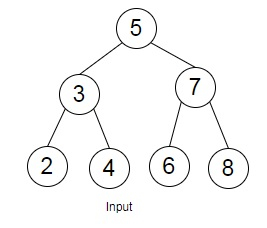# Check if a triplet with given sum exists in BST in Python

Suppose, we are provided with a Binary Search Tree (BST) that contains integer values, and a number 'total'. We have to find out if there are any group of three elements in the provided BST where the addition of the three elements is equal to the supplied 'total' value.

So, if the input is liketotal = 12, then the output will be True.

To solve this, we will follow these steps −

• temp_list := a new list initialized with zero
• inorder traverse the tree and put it in temp_list
• for i in range 0 to (size of temp_list - 2), increase by 1, do
• left := i + 1
• right := size of temp_list - 1
• while left < right, do
• if temp_list[i] + temp_list[left] + temp_list[right] is same as sum, then
• return True
• otherwise when temp_list[i] + temp_list[left] + temp_list[right] < sum is non-zero, then
• left := left + 1
• otherwise,
• right := right - 1
• return False

## Example

Let us see the following implementation to get better understanding −

Live Demo

class TreeNode:
def __init__(self, value):
self.value = value
self.right = None
self.left = None
def traverse_inorder(tree_root, inorder):
if tree_root is None:
return
traverse_inorder(tree_root.left, inorder)
inorder.append(tree_root.value)
traverse_inorder(tree_root.right, inorder)
def solve(tree_root, sum):
temp_list = 
traverse_inorder(tree_root, temp_list)
for i in range(0, len(temp_list) - 2, 1):
left = i + 1
right = len(temp_list) - 1
while(left < right):
if temp_list[i] + temp_list[left] + temp_list[right] == sum:
return True
elif temp_list[i] + temp_list[left] + temp_list[right] < sum:
left += 1
else:
right -= 1
return False
tree_root = TreeNode(5)
tree_root.left = TreeNode(3)
tree_root.right = TreeNode(7)
tree_root.left.left = TreeNode(2)
tree_root.left.right = TreeNode(4)
tree_root.right.left = TreeNode(6)
tree_root.right.right = TreeNode(8)
print(solve(tree_root, 12))

## Input

tree_root = TreeNode(5)
tree_root.left = TreeNode(3)
tree_root.right = TreeNode(7)
tree_root.left.left = TreeNode(2)
tree_root.left.right = TreeNode(4)
tree_root.right.left = TreeNode(6)
tree_root.right.right = TreeNode(8)
12

## Output

True